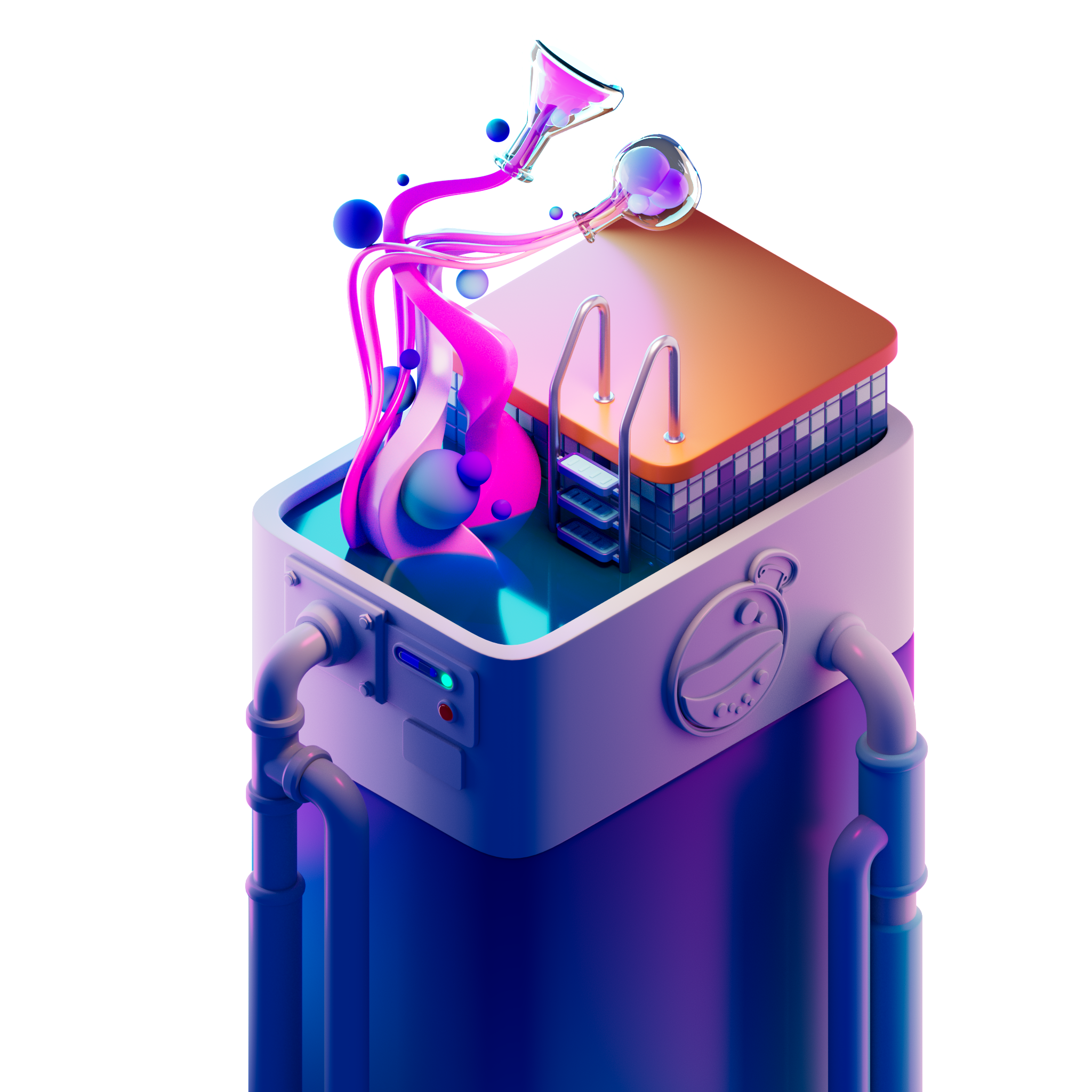0% is complete

# What is GAMM?

Last updated on
Apr 7, 2023
Explanation of LP tokens.• GAMM LP-tokens are derivative placement holders to represent your portion of a pool. Let’s dive into what it means to own a portion of a pool.
• Osmosis is a decentralized exchange. It functions on the basis of the community adding pairs or pools of assets together to allow for trading in the market. The market here refers to everyone who trades on the Osmosis platform.
• When you add your assets into a pool, you are putting your assets on the market as liquidity to be used for trades. That means if you add a combination of OSMO and ATOM together to pool 1, at any time of day someone can come by to take some of your OSMO out and give you ATOM in exchange. This is what happens during a swap. The user is swapping with every pool owner proportionately to their total percentage of the pool owned.
• Now to the role of GAMM. Simply put, GAMM is meant to represent your held tokens in a pool vs the rest of the LP holders.

For example, when you add liquidity of x ATOM and y OSMO to Pool 1, you are given an amount of GAMM LP-token that represents the value of x and y combined within that specific pool (Pool 1).

GAMM has no realizable dollar value as its use case is currently limited to this representation role and, in reference to the example, since the price of both ATOM and OSMO are changing constantly with the flow of the market, the dollar value of the GAMM token is in a constantly changing as well.

In our example, Pool 1 is a “balancer-type” pool which follows a specific formula to try and keep an equilibrium. That formula looks like (x*y=k). *Note: This is a constant product formula where the integer of k does not change.
• Someone comes along and swaps 1 ATOM. To determine how many OSMO are given back to the trader, we use the constant product formula y*4=90. To find out how many OSMO equal the value of 1 ATOM, we take that number 90 and divide by the new number of ATOM, which is 4. 90/4=y. Y=22.5. 22.5 is the new number of OSMO that should be in the pool in comparison with the new number of ATOM, and so the trader will get the difference in OSMO taken out of the pool. They get this back so that the pool equation remains true that x*y=k. Since y=22.5 and there were 30 before, the trader will be getting back 7.5 OSMO in exchange for 1 ATOM. 30-22.5=7.5.
• Now the pool equation is 22.5*4=90. That number 90 will never change, but as you add or remove either asset, both x and y will adjust themselves to be a multiplication answer of 90. That is what determines the trading value between assets in a pool.